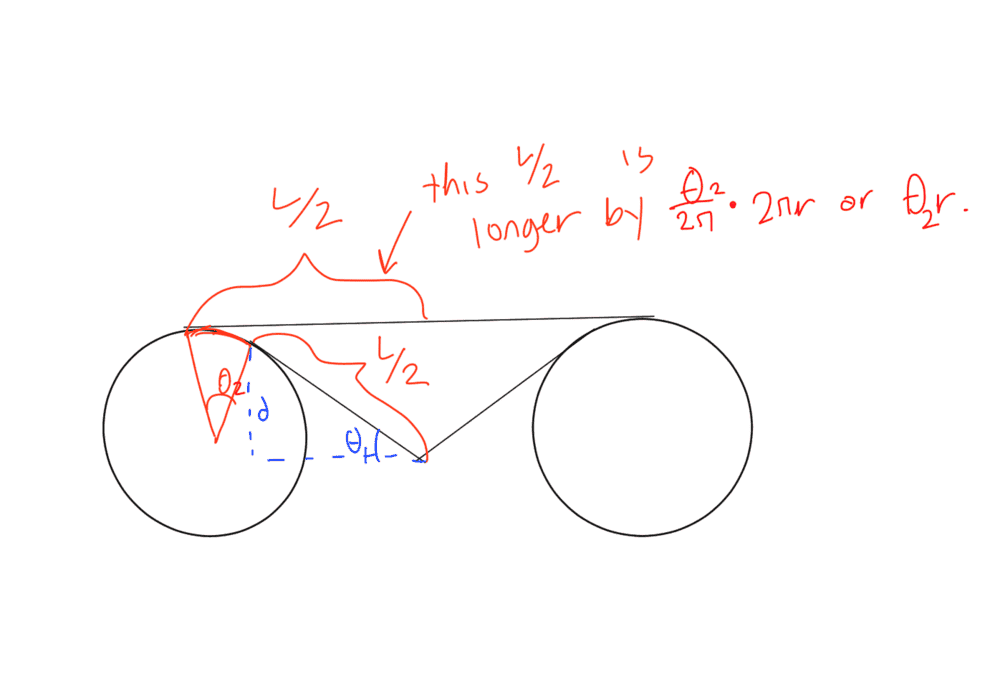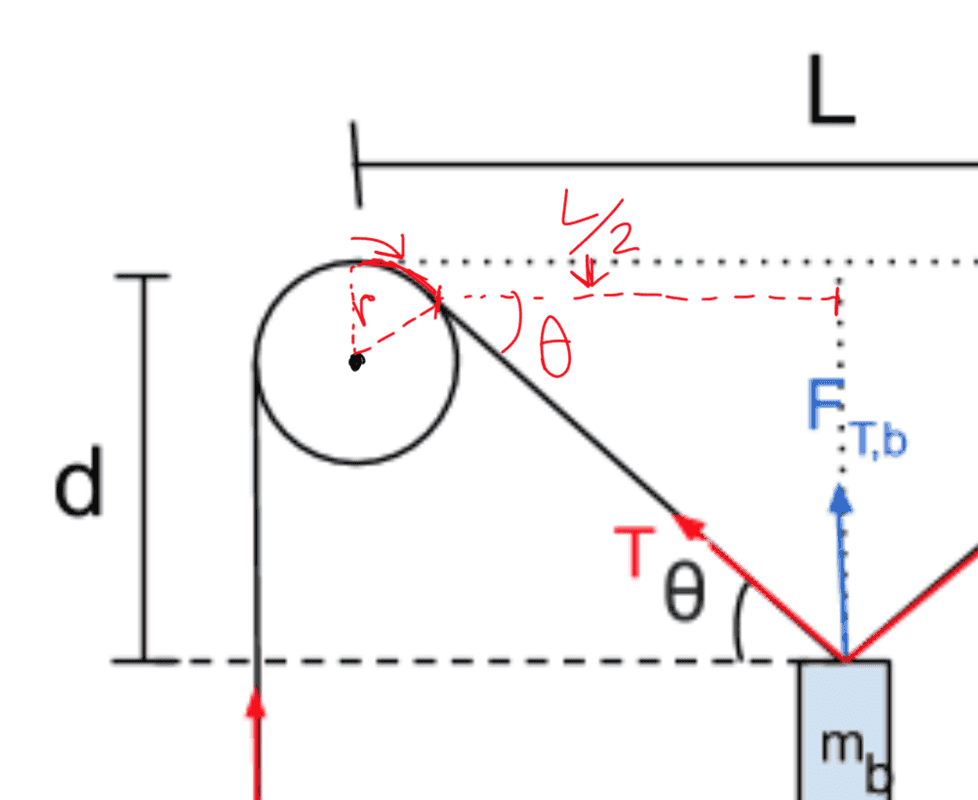# Length of a string over a 2 pulley system

Homework Statement:
How does L change as mb gets heavier?
Relevant Equations:
d=m(L/2)/(sqrt((2M)^2-(m)^2))
Hello all,
I am working on a lab report for physics, and am a bit stuck on one aspect. The basic layout of the pulley system is attached. (This is a diagram I made, so if there are any inaccuracies in this one either, I am happy to fix it.So, I mostly understand the lab. My TA said, though, that L/2 changes as more weight is added onto mb.
Here is my attempt at understanding why:Is this accurate? I just wanted to check.

Homework Statement: How does L change as mb gets heavier?
Homework Equations: d=m(L/2)/(sqrt((2M)^2-(m)^2))

Hello all,
I am working on a lab report for physics, and am a bit stuck on one aspect. The basic layout of the pulley system is attached. (This is a diagram I made, so if there are any inaccuracies in this one either, I am happy to fix it.So, I mostly understand the lab. My TA said, though, that L/2 changes as more weight is added onto mb.
Here is my attempt at understanding why:
View attachment 252745
Is this accurate? I just wanted to check.

What is mb?

mb is the weight in the middle, the diagram shows it if you can see the diagrammb is the weight in the middle, the diagram shows it if you can see the diagramAre the string endpoints fixed to the tops of the pulleys?

Are the string endpoints fixed to the tops of the pulleys?
No, it's one long string that has 3 weights on it, and goes through both the pulleys. Would the amount of string lost on the inside by wrapping over the pulley be made up for by pulling more string over?

haruspex
Homework Helper
Gold Member
2020 Award
No, it's one long string that has 3 weights on it, and goes through both the pulleys. Would the amount of string lost on the inside by wrapping over the pulley be made up for by pulling more string over?
Consider the point where the string touches the (rotated) pulley. How far is it from a vertical line through the pulley's centre? How far is it from a vertical line through the mass? What equation does that allow you to write?

If I'm interpreting you right, the point where the string touches the pulley to the center should just be the radius of the pulley? The distance to the mass would be the changed L/2? I tried drawing out a diagram.I am a bit confused on where to go from here, however?

haruspex